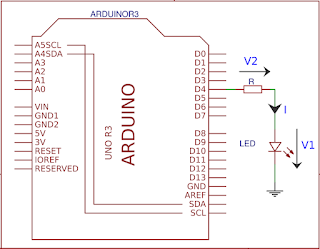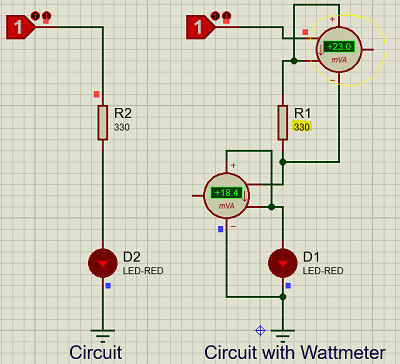## Pages

### When and Where we can use resistors to step down voltages

We've already discussed some points on Buck Converters, Linear Regulators and LDOs. (Linear Drop Out Regulators) Reading following posts will give you little more knowledge on other options to step down the voltage.

When it comes to stepping down/reducing voltage first thing that may come to your mind would be resistors. True, there are occasions that we can use resistors, And there are occasions that we can't. Lets have a look at what they are. Following example is from my previous example of Arduino Blink.

### "Calculating the current limiting resistor value

Generally we put 270 Ohms or 330 Ohms resistors in series when selecting a current limiting resistor. However most people does not have an idea about selecting the resistor. Understanding the concept behind this will help you to select current limiting resistors for different scenarios. Also it is really important to verify that heat emission must be lesser than the wattage of the resistorCurrent Limiting Resistor, Arduino Blink{In a series circuit, current through each component is same and, the voltage across the circuit is the sum of voltages across each component.}

V1 = 2.2V (assuming 2.2V rated LED)

Therefore,
V2 = 2.8V  (Above equation, Kirchhoff's Voltage Law)
Applying Ohms Law for the R resistor,

Since 280 Ohms resistors are not available we can select either 270 Ohms or 330 Ohms resistors. However this value can be changed depending on the current LED draws.(depend on the colour, size etc of the LED)"

Lets see the power dissipation calculation in Resistor and the LED,We can simulate the result using Proteus. (There is a very small difference in the values due to LED current I've assumed in above calculation)Power dissipation Simulation
• If we take the above example efficiency is only about 44% {=18.7/(18.7+23.8)}
• And resistor must have the capability to dissipate the wattage of 23.8 mW

Lets take another application for the below specification,

• Supply Voltage = 24 V
• Lamp Voltage = 12 V
• Lamp Rated Current = 6 Ohms

To reduce the voltage resistor should also be 6 Ohms,Resistor Voltage Reduction

As per this circuit,

• Efficiency is only 50%
• Since resistor should have the capability to dissipate 23.3 W it has to be a considerably large one.

Conclusion
• Resistor Voltage reduction options are not efficient.
• If the power dissipation is high (Load draws more current/Voltage drop is high) resistors has to be larger.

Though we have not proved, following rules also has to be considered

• If the supply voltage changes, output voltage also will change.
• If the load current is not constant, output voltage also varies.
Linear Regulators, Buck Converters and LDOs are much more better than resistor voltage reducing circuits. However the main disadvantage of them compared to resistors is that they may generate harmonics/noises due to high speed switching. (Non-Linearity) Generally resistors are good only when the supply voltage is constant, Load current is constant, Load requires a very minimum current and the voltage drop required is very low.